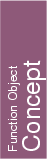## Kernel::Equal_2

A model for this must provide the following operations. For all of them fo(x,y) returns true iff x and y are equal.

bool
 fo.operator() ( Kernel::Point_2 x, Kernel::Point_2 y)

bool
 fo.operator() ( Kernel::Vector_2 x, Kernel::Vector_2 y)

bool
 fo.operator() ( Kernel::Direction_2 x, Kernel::Direction_2 y)

bool fo.operator() ( Kernel::Line_2 x, Kernel::Line_2 y)

bool fo.operator() ( Kernel::Ray_2 x, Kernel::Ray_2 y)

bool
 fo.operator() ( Kernel::Segment_2 x, Kernel::Segment_2 y)

bool
 fo.operator() ( Kernel::Circle_2 x, Kernel::Circle_2 y)

bool
 fo.operator() ( Kernel::Triangle_2 x, Kernel::Triangle_2 y)

bool
 fo.operator() ( Kernel::Iso_rectangle_2 x, Kernel::Iso_rectangle_2 y)